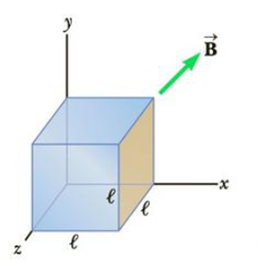Chapter 20, Problem 7P

Chapter
Section
Textbook Problem

A cube of edge length ℓ = 2.5 cm is positioned as shown in Figure P20.7. There is a uniform magnetic field throughout the region with components Bx = +5.0 T, By = +4.0 T, and Bz = +3.0 T. (a) Calculate the flux through the shaded face of the cube. (b) What is the total flux emerging from the volume enclosed by the cube (i.e., the total flux through all six faces)?Figure P20.7

(a)

To determine
The total flux through the shaded face of the cube.

Explanation

Given Info:

The edge length of the cube is, l=2.5cm .

The components of the uniform magnetic field is,

Bx=+5.0T

By=+4.0T

Bz=+3.0T

Consider the shaded area as A,

The magnetic flux through the area A will be the product of the perpendicular magnetic field component to the shaded area with the area of the shaded region A.

From the figure, the perpeticular magnetic field component to the shaded area is Bx .

Thus,

ϕ=BxA

• A is the area of the shaded region
• Bx is the x-component of the uniform magnetic field

The area of the shaded region is,

A=l2

Thus,

Formula to calculate the flux through the shaded flux of the cube is,

ϕ=Bx(l2)

Substitute 5

(b)

To determine
The total flux emerging from the volume enclosed by the cube.

Still sussing out bartleby?

Check out a sample textbook solution.

See a sample solution

The Solution to Your Study Problems

Bartleby provides explanations to thousands of textbook problems written by our experts, many with advanced degrees!

Get Started